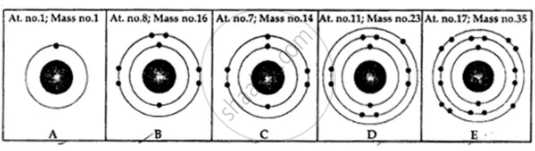# State the Number of Neutrons in Each of the Atoms a to E. Also State Which of the Atoms a to E is a Metal. - Chemistry

Numerical

State the number of neutrons in each of the atoms A to E. Also state which of the atoms A to E is a metal.#### Solution

Number of neutrons = A – Z
= Mass number – Atomic number
A. Number of neutrons =1 – 1 = 0
B. Number of neutrons = 16 – 8 = 8
C. Number of neutrons = 14 – 7 = 7
D. Number of neutrons = 23 – 11 = 12 (2, 8, 1 metal)
E. Number of neutrons = 35 – 17 = 18

Concept: Sub-atomic Particles of Atom - Neutrons (N)
Is there an error in this question or solution?

#### APPEARS IN

Viraf J. Dalal Class 9 Simplified ICSE Chemistry
Chapter 4 Atomic Structure & Chemical Bonding
Atomic Structure | Q 5